Welcome, Guest. Please Login or Register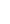HomeHelpSearchLoginRegisterSimviation Forum › Current Flight Simulator Series › Flight Simulator X › Programming doorsPages: 1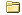Programming doors (Read 1615 times)Nov 21st, 2011 at 4:54pmcoalpedlar   Offline Colonel Like My Aircraft heavy; my women light! Commonwealth of  Virginia USA Gender: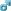Posts: 161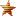Somewhere on here I saw a file on how to program door locations in cfg files for addon aircraft to enable the jetways to operate.I can't find it for the life of me.anyone know where it is???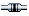IP LoggedReply #1 - Nov 21st, 2011 at 5:12pmBoikat   Offline Colonel Hello! NW Loueezianner Gender:Posts: 2978It's probably the [exits] entry in the .cfg.  If the addon does not have an [exits] section, you can probably copy/past/modify/tweak the [exits] from a similar sized default airliner."I reject your reality, and substitute my own" Adam Savage, MythbustersIP LoggedReply #2 - Nov 21st, 2011 at 6:28pmcoalpedlar   Offline Colonel Like My Aircraft heavy; my women light! Commonwealth of  Virginia USA Gender:Posts: 161Thank you.Yes, I know that.  But, if it's not the same dimensions as another aircraft with exits it won't work, now will it?I'm looking for the file with the explanation of coordinates.  Do you know where it is?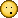IP LoggedReply #3 - Nov 22nd, 2011 at 7:35am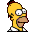striker   Offline Colonel Don't read this. Read my post. Wilmington, NC Posts: 110From another site: Quote:With FSX and Jetways. I do feel it would be much easier for many if those posting aircraft would be helpful to include the Jetways in the aircraft.cfg before posting.thanks for your efforts with bringing aircraft to us.Will be adding Jetways as I go with edit, putting them in sequence best I can, with a rough idea of what figures below to use on the aircraft config. The figures I use may be wrong but try them, as I am no expert and it takes time, aircraft creep does not help on some. They are set for the front door, as I did not like seeing jetway split in bits as it stretches to meet aircraft. Some aircraft vary between models and who put them together. I have tried to set these figures so that if one opens the aircraft door it is inside the jetway and not sticking out the side and to put the height of one at door level. One will find the middle door figures, read below.Just one thing about Jetways. I find it better to put the size of aircraft in right jetway being it a small, medium or heavy bay for them to work, especially if your going directly to airport then expecting them to work. So if you have a heavy aircraft put it in a heavy jetway spot not in a small. One other thing is if the aircraft is to far forward may also stop jetway from working, most of it depends on type of parking bay. I have also done a few smaller jets that probably would be better with stairs.Would be nice to have other buttons in FSX giving one a choice when parked to select front door, middle door exits for jetway.To change your aircraft.cfg file with those below to make Jetways work Browse down your aircraft config file to where exits are and replace with section of type for the aircraft.Found that if one changes "number_of_exits = " from 4, 3 to 2 one may find that all doors may not open. Always best to try aircraft before changing aircraft config to see what doors do open.both front and middle doors done To change from front door to rear middle door just swap figures as I have done with A350-1000 Air Caraibes. Do not swap with aircraft that do not have middle door, usually the smaller aircraft.A350-1000 Air Caraibes Front door Jetway[exits]number_of_exits = 4exit.0 = 0.4, 95.00, -4.5, 8.5, 0 //openclose rate percent per second, longitudinal, lateral, vertical positions from datum (feet), type (0=Main 1=Cargo 2=Emergency)exit.1 = 0.4, 48.30, -4.5, 8.5, 2 //openclose rate percent per second, longitudinal, lateral, vertical positions from datum (feet), type (0=Main 1=Cargo 2=Emergency)exit.2=0.4, -18.40, 7.0, 0.7, 1 //openclose rate percent per second, longitudinal, lateral, vertical positions from datum (feet), type (0=Main 1=Cargo 2=Emergency)A350-1000 Air Caraibes, BA Middle door jetway[exits]number_of_exits = 4exit.0 = 0.4, 48.30, -4.5, 11.5, 0 //openclose rate percent per second, longitudinal, lateral, vertical positions from datum (feet), type (0=Main 1=Cargo 2=Emergency)exit.1 = 0.4, 95.00, -4.5, 11.5, 2 //openclose rate percent per second, longitudinal, lateral, vertical positions from datum (feet), type (0=Main 1=Cargo 2=Emergency)exit.2=0.4, -18.40, 7.0, 0.7, 1 //openclose rate percent per second, longitudinal, lateral, vertical positions from datum (feet), type (0=Main 1=Cargo 2=Emergency)Remember to set scenery complexity to very high to make Jetways visible.To activate Jetways press keyboard Ctrl+J================================================== ===================================================== ===================================================== ===Brand A both front and middle doors doneA350-900 Singapore Airlines[exits]number_of_exits = 4exit.0 = 0.4, 85.00, -4.5, 11.5, 0 //openclose rate percent per second, longitudinal, lateral, vertical positions from datum (feet), type (0=Main 1=Cargo 2=Emergency)exit.1 = 0.4, 45.00, -4.5, 11.5, 2 //openclose rate percent per second, longitudinal, lateral, vertical positions from datum (feet), type (0=Main 1=Cargo 2=Emergency)exit.2=0.4, -18.40, 7.0, 0.7, 1 //openclose rate percent per second, longitudinal, lateral, vertical positions from datum (feet), type (0=Main 1=Cargo 2=Emergency)A350-1000 Air Caraibes, BA[exits]number_of_exits = 4exit.0 = 0.4, 95.00, -4.5, 11.5, 0 //openclose rate percent per second, longitudinal, lateral, vertical positions from datum (feet), type (0=Main 1=Cargo 2=Emergency)exit.1 = 0.4, 48.30, -4.5, 11.5, 2 //openclose rate percent per second, longitudinal, lateral, vertical positions from datum (feet), type (0=Main 1=Cargo 2=Emergency)exit.2=0.4, -18.40, 7.0, 0.7, 1 //openclose rate percent per second, longitudinal, lateral, vertical positions from datum (feet), type (0=Main 1=Cargo 2=Emergency)A360 Air France[exits]number_of_exits = 2exit.0 = 0.4, 52.00, -7.0, 2.00, 0 //openclose rate percent per second, longitudinal, lateral, vertical positions from datum (feet), type (0=Main 1=Cargo 2=Emergency)exit.1 = 0.4, 52.00, -7.0, 2.00, 1 //openclose rate percent per second, longitudinal, lateral, vertical positions from datum (feet), type (0=Main 1=Cargo 2=Emergency)exit.2 = 0.4, -42.30, 9.0, -7, 2 //openclose rate percent per second, longitudinal, lateral, vertical positions from datum (feet), type (0=Main 1=Cargo 2=Emergency)B700 Qantas, KLM[exits]number_of_exits = 4exit.0 = 0.4, 89.00, -7.0, 13.30, 0 //openclose rate percent per second, longitudinal, lateral, vertical positions from datum (feet), type (0=Main 1=Cargo 2=Emergency)exit.1 = 0.4, 52.00, -7.0, 13.30, 1 //openclose rate percent per second, longitudinal, lateral, vertical positions from datum (feet), type (0=Main 1=Cargo 2=Emergency)exit.2 = 0.4, -42.30, 9.0, -7, 2 //openclose rate percent per second, longitudinal, lateral, vertical positions from datum (feet), type (0=Main 1=Cargo 2=Emergency)B767-300 AA[exits]number_of_exits = 2exit.0= 0.10, 62.6, -7.0, 7.0, 0 //openclose rate percent per second, longitudinal, lateral, vertical positions from datum (feet), type (0=Main 1=Cargo 2=Emergency)exit.1= 0.09, -21.5, 5.5, -6.8, 1 //openclose rate percent per second, longitudinal, lateral, vertical positions from datum (feet), type (0=Main 1=Cargo 2=Emergency)B787-8 Air Canada[exits]number_of_exits = 4exit.0 = 0.4, 77.30, -4.5, 12.5, 0 //openclose rate percent per second, longitudinal, lateral, vertical positions from datum (feet), type (0=Main 1=Cargo 2=Emergency)exit.1 = 0.4, 48.00, -4.5, 12.5, 1 //openclose rate percent per second, longitudinal, lateral, vertical positions from datum (feet), type (0=Main 1=Cargo 2=Emergency)exit.2=0.4, -18.40, 7.0, 0.7, 2 //openclose rate percent per second, longitudinal, lateral, vertical positions from datum (feet), type (0=Main 1=Cargo 2=Emergency)B787-9 Qantas[exits]number_of_exits = 4exit.0 = 0.4, 86.50, -4.5, 12.5, 0 //openclose rate percent per second, longitudinal, lateral, vertical positions from datum (feet), type (0=Main 1=Cargo 2=Emergency)exit.1 = 0.4, 46.50, -4.5, 12.5, 1 //openclose rate percent per second, longitudinal, lateral, vertical positions from datum (feet), type (0=Main 1=Cargo 2=Emergency)exit.2=0.4, -18.40, 7.0, 0.7, 2 //openclose rate percent per second, longitudinal, lateral, vertical positions from datum (feet), type (0=Main 1=Cargo 2=Emergency)B787-900 Cargo FedEx[exits]number_of_exits = 4exit.0 = 0.4, 86.50, -4.5, 12.5, 0 //openclose rate percent per second, longitudinal, lateral, vertical positions from datum (feet), type (0=Main 1=Cargo 2=Emergency)exit.1 = 0.4, 86.50, -4.5, 12.5, 1 //openclose rate percent per second, longitudinal, lateral, vertical positions from datum (feet), type (0=Main 1=Cargo 2=Emergency)exit.2=0.4, -18.40, 7.0, 0.7, 2 //openclose rate percent per second, longitudinal, lateral, vertical positions from datum (feet), type (0=Main 1=Cargo 2=Emergency)B787 Super-11 Air France, Emirates[exits]number_of_exits = 4exit.0 = 0.4, 108.0, -7.0, 16.3, 0 //openclose rate percent per second, longitudinal, lateral, vertical positions from datum (feet), type (0=Main 1=Cargo 2=Emergency)exit.1 = 0.4, 49.3, -7.0, 16.3, 1 //openclose rate percent per second, longitudinal, lateral, vertical positions from datum (feet), type (0=Main 1=Cargo 2=Emergency)exit.2 = 0.4, -42.3, 9.0, -7, 2 //openclose rate percent per second, longitudinal, lateral, vertical positions from datum (feet), type (0=Main 1=Cargo 2=Emergency)C 110 Air Canada[exits]number_of_exits = 2exit.0 = 0.4, 40.50, -7.0, 2.00, 0 //openclose rate percent per second, longitudinal, lateral, vertical positions from datum (feet), type (0=Main 1=Cargo 2=Emergency)exit.1 = 0.4, 40.50, -7.0, 2.00, 1 //openclose rate percent per second, longitudinal, lateral, vertical positions from datum (feet), type (0=Main 1=Cargo 2=Emergency)exit.2 = 0.4, -42.30, 9.0, -7, 2 //openclose rate percent per second, longitudinal, lateral, vertical positions from datum (feet), type (0=Main 1=Cargo 2=Emergency)C 130 Lufthansa Regional-Eurowings[exits]number_of_exits = 2exit.0 = 0.4, 46.00, -7.0, 2.00, 0 //openclose rate percent per second, longitudinal, lateral, vertical positions from datum (feet), type (0=Main 1=Cargo 2=Emergency)exit.1 = 0.4, 46.00, -7.0, 2.00, 1 //openclose rate percent per second, longitudinal, lateral, vertical positions from datum (feet), type (0=Main 1=Cargo 2=Emergency)exit.2 = 0.4, -42.30, 9.0, -7, 2 //openclose rate percent per second, longitudinal, lateral, vertical positions from datum (feet), type (0=Main 1=Cargo 2=Emergency)================================================== ===================================================== ===================================================== ===Brand B Few below with front and middle door setA330 House AC, Cp, SAS[exits]number_of_exits = 4exit.0 = 0.4, 71.00, -6.0, 9.5, 0 //openclose rate percent per second, longitudinal, lateral, vertical positions from datum (feet), type (0=Main 1=Cargo 2=Emergency)exit.1 = 0.4, 54.0, 8.0, -5.5, 1 //openclose rate percent per second, longitudinal, lateral, vertical positions from datum (feet), type (0=Main 1=Cargo 2=Emergency)exit.2 = 0.4, -42.30, 9.0, -7, 2 //openclose rate percent per second, longitudinal, lateral, vertical positions from datum (feet), type (0=Main 1=Cargo 2=Emergency)B700-200 BA, BA Landor[exits]number_of_exits = 4exit.0 = 0.4, 72.1, -7.0, 8.00,0 //openclose rate percent per second, longitudinal, lateral, vertical positions from datum (feet), type (0=Main 1=Cargo 2=Emergency)exit.1 = 0.4, 39.5, -7.0, 8.00, 1 //openclose rate percent per second, longitudinal, lateral, vertical positions from datum (feet), type (0=Main 1=Cargo 2=Emergency)exit.2 = 0.4, -42.30, 9.0, -7, 2 //openclose rate percent per second, longitudinal, lateral, vertical positions from datum (feet), type (0=Main 1=Cargo 2=Emergency)B737-600 Austrian Airlines[exits]number_of_exits = 2exit.0 = 0.4, 34.0, -5.0, 3.0, 0 //openclose rate percent per second, longitudinal, lateral, vertical positions from datum (feet), type (0=Main 1=Cargo 2=Emergency)exit.1 = 0.4, -23.0, 4.5, -3.0, 1 //openclose rate percent per second, longitudinal, lateral, vertical positions from datum (feet), type (0=Main 1=Cargo 2=Emergency)B737-700 Virgin Blue[exits]number_of_exits = 2exit.0 = 0.4, 37.5, -5.0, 3.0, 0 //openclose rate percent per second, longitudinal, lateral, vertical positions from datum (feet), type (0=Main 1=Cargo 2=Emergency)exit.1 = 0.4, -28.0, 4.5, -3.0, 1 //openclose rate percent per second, longitudinal, lateral, vertical positions from datum (feet), type (0=Main 1=Cargo 2=Emergency)B737-800 Air India AXX, Hapag Lloyd OC, Sterling[exits]number_of_exits = 2exit.0 = 0.4, 48.0, -5.0, 3.0, 0 //openclose rate percent per second, longitudinal, lateral, vertical positions from datum (feet), type (0=Main 1=Cargo 2=Emergency)exit.1 = 0.4, -28.0, 4.5, -3.0, 1 //openclose rate percent per second, longitudinal, lateral, vertical positions from datum (feet), type (0=Main 1=Cargo 2=Emergency)B737-900ER Avianca[exits]number_of_exits = 2exit.0 = 0.4, 51.0, -5.0, 2.5, 0 //openclose rate percent per second, longitudinal, lateral, vertical positions from datum (feet), type (0=Main 1=Cargo 2=Emergency)exit.1 = 0.4, -38.5, 4.5, -3.0, 1 //openclose rate percent per second, longitudinal, lateral, vertical positions from datum (feet), type (0=Main 1=Cargo 2=Emergency)exit.2 = 0.4, 33.5, 3.5, -4.0, 2 //openclose rate percent per second, longitudinal, lateral, vertical positions from datum (feet), type (0=Main 1=Cargo 2=Emergency)B747SP NZ[exits]number_of_exits = 2exit.0 = 0.4, -42.30, -9.5, 9.0, 0 //openclose rate percent per second, longitudinal, lateral, vertical positions from datum (feet), type (0=Main 1=Cargo 2=Emergency)exit.1 = 0.4, -42.30, -9.5, 9.0, 1 //openclose rate percent per second, longitudinal, lateral, vertical positions from datum (feet), type (0=Main 1=Cargo 2=Emergency)exit.2 = 0.4, -42.30, 9.0, -7, 2 //openclose rate percent per second, longitudinal, lateral, vertical positions from datum (feet), type (0=Main 1=Cargo 2=Emergency)B747-100 Lan Chile[exits]number_of_exits = 4exit.0 = 0.4, -16.00, -9.0, 9.5, 0 //openclose rate percent per second, longitudinal, lateral, vertical positions from datum (feet), type (0=Main 1=Cargo 2=Emergency)exit.1 = 0.4, -44.70, -9.0, 9.5, 1 //openclose rate percent per second, longitudinal, lateral, vertical positions from datum (feet), type (0=Main 1=Cargo 2=Emergency)exit.2 = 0.4, -42.30, 9.0, -7, 2 //openclose rate percent per second, longitudinal, lateral, vertical positions from datum (feet), type (0=Main 1=Cargo 2=Emergency)B747-200GE Air Force One[exits]number_of_exits = 4exit.0 = 0.4, -16.00, -9.0, 9.5, 0 //openclose rate percent per second, longitudinal, lateral, vertical positions from datum (feet), type (0=Main 1=Cargo 2=Emergency)exit.1 = 0.4, -44.70, -9.0, 9.5, 1 //openclose rate percent per second, longitudinal, lateral, vertical positions from datum (feet), type (0=Main 1=Cargo 2=Emergency)exit.2 = 0.4, -42.30, 9.0, -7, 2 //openclose rate percent per second, longitudinal, lateral, vertical positions from datum (feet), type (0=Main 1=Cargo 2=Emergency)B747-200 Varig[exits]number_of_exits = 4exit.0 = 0.4, -24.70, -9.0, 9.5, 0 //openclose rate percent per second, longitudinal, lateral, vertical positions from datum (feet), type (0=Main 1=Cargo 2=Emergency)exit.1 = 0.4, -54.70, -9.0, 9.5, 1 //openclose rate percent per second, longitudinal, lateral, vertical positions from datum (feet), type (0=Main 1=Cargo 2=Emergency)exit.2 = 0.4, -42.30, 9.0, -7, 2 //openclose rate percent per second, longitudinal, lateral, vertical positions from datum (feet), type (0=Main 1=Cargo 2=Emergency)   &&IP LoggedReply #4 - Nov 22nd, 2011 at 7:38amstriker   Offline Colonel Don't read this. Read my post. Wilmington, NC Posts: 110Quote:B757-200 House, IceAir, Airtran[exits]number_of_exits = 2exit.0 = 0.1, 62.0, -5.5, 8.0, 0 //openclose rate percent per second, longitudinal, lateral, vertical positions from datum (feet), type (0=Main 1=Cargo 2=Emergency)exit.1 = 0.1, 33.20, -5.5, 8.0, 1 //openclose rate percent per second, longitudinal, lateral, vertical positions from datum (feet), type (0=Main 1=Cargo 2=Emergency)B767-300 ANZ[exits]number_of_exits = 2exit.0 = 0.1, 62.0, -5.5, 7.5, 0 //openclose rate percent per second, longitudinal, lateral, vertical positions from datum (feet), type (0=Main 1=Cargo 2=Emergency)exit.1 = 0.1, 64.5, 5.85, 7.5, 1 //openclose rate percent per second, longitudinal, lateral, vertical positions from datum (feet), type (0=Main 1=Cargo 2=Emergency)B767-300ER Delta[exits]number_of_exits = 2exit.0 = 0.1, 73.0, -5.5, 7.5, 0 //openclose rate percent per second, longitudinal, lateral, vertical positions from datum (feet), type (0=Main 1=Cargo 2=Emergency)exit.1 = 0.1, 73.0, -5.5, 7.5, 1 //openclose rate percent per second, longitudinal, lateral, vertical positions from datum (feet), type (0=Main 1=Cargo 2=Emergency)B777-200ER Saudi Arabian, British Airways(Union,Wings), Alitalia[exits]number_of_exits = 4exit.0 = 0.4, 72.6, -7.0, 8.00,0 //openclose rate percent per second, longitudinal, lateral, vertical positions from datum (feet), type (0=Main 1=Cargo 2=Emergency)exit.1 = 0.4, 39.5, -7.0, 8.00, 1 //openclose rate percent per second, longitudinal, lateral, vertical positions from datum (feet), type (0=Main 1=Cargo 2=Emergency)exit.2 = 0.4, -42.30, 9.0, -7, 2 //openclose rate percent per second, longitudinal, lateral, vertical positions from datum (feet), type (0=Main 1=Cargo 2=Emergency)B777-200 British Airways (Landor)number_of_exits = 4exit.0 = 0.4, 72.1, -7.0, 8.00,0 //openclose rate percent per second, longitudinal, lateral, vertical positions from datum (feet), type (0=Main 1=Cargo 2=Emergency)exit.1 = 0.4, 40.5, -7.0, 8.00, 1 //openclose rate percent per second, longitudinal, lateral, vertical positions from datum (feet), type (0=Main 1=Cargo 2=Emergency)exit.2 = 0.4, -42.30, 9.0, -7, 2 //openclose rate percent per second, longitudinal, lateral, vertical positions from datum (feet), type (0=Main 1=Cargo 2=Emergency)================================================== ===================================================== ===================================================== ===Brand CA318-100 CFM Iberia[exits]number_of_exits = 2exit.0 = 0.4, 27.00, -6.0, 2.0, 0 //openclose rate percent per second, longitudinal, lateral, vertical positions from datum (feet), type (0=Main 1=Cargo 2=Emergency)exit.1 = 0.4, -33.50, 5.85, 0.0, 1 //openclose rate percent per second, longitudinal, lateral, vertical positions from datum (feet), type (0=Main 1=Cargo 2=Emergency)===================================Brand DA380-800 Qantas, Palestinian, KLM, Ethiopian, AF, Continental, UPs[exits]number_of_exits = 4exit.0 = 0.4, 102.00, -7.0, 14.50, 0 //openclose rate percent per second, longitudinal, lateral, vertical positions from datum (feet), type (0=Main 1=Cargo 2=Emergency)exit.1 = 0.4, 69.00, -7.0, 14.50, 1 //openclose rate percent per second, longitudinal, lateral, vertical positions from datum (feet), type (0=Main 1=Cargo 2=Emergency)exit.2 = 0.4, 55.00, -7.0, 20.50, 2 //openclose rate percent per second, longitudinal, lateral, vertical positions from datum (feet), type (0=Main 1=Cargo 2=Emergency)=============================B707 Pan Am, Baremetal Cargo, Argentinas, Aerolineas Argentinas Cargo, Ecuatoriana Cargo, Ecuatoriana, Western, Avianca, Air France[exits]number_of_exits = 2exit.0 = 0.4, 66.00, -7.0, 1.50, 0 //openclose rate percent per second, longitudinal, lateral, vertical positions from datum (feet), type (0=Main 1=Cargo 2=Emergency)exit.1 = 0.4, 52.00, -7.0, 2.00, 1 //openclose rate percent per second, longitudinal, lateral, vertical positions from datum (feet), type (0=Main 1=Cargo 2=Emergency)exit.2 = 0.4, -42.30, 9.0, -7, 2 //openclose rate percent per second, longitudinal, lateral, vertical positions from datum (feet), type (0=Main 1=Cargo 2=Emergency)==================================B727-100 Delta, Fedex, Piedmont, NWA, United[exits]number_of_exits = 4exit.0 = 0.4, 54.50, -6.0, 5.5, 0 //openclose rate percent per second, longitudinal, lateral, vertical positions from datum (feet), type (0=Main 1=Cargo 2=Emergency)exit.1 = 0.4, 54.50, -6.0, 5.5, 1 //openclose rate percent per second, longitudinal, lateral, vertical positions from datum (feet), type (0=Main 1=Cargo 2=Emergency)exit.2 = 0.4, -42.30, 9.0, -7, 2 //openclose rate percent per second, longitudinal, lateral, vertical positions from datum (feet), type (0=Main 1=Cargo 2=Emergency)==================================BAe142-600 Qantas, British Aerospace, Lufthansa, British Airways[exits]number_of_exits = 2exit.0 = 0.4, 33.0, -5.0, 0.7, 0 //openclose rate percent per second, longitudinal, lateral, vertical positions from datum (feet), type (0=Main 1=Cargo 2=Emergency)exit.1 = 0.4, -28.0, 4.5, -3.0, 1 //openclose rate percent per second, longitudinal, lateral, vertical positions from datum (feet), type (0=Main 1=Cargo 2=Emergency)===================================DC-10-30 Swissair, Singapore Airlines[exits]number_of_exits = 4exit.0 = 0.4, 79.0, -8.50, 13.0, 0 //openclose rate percent per second, longitudinal, lateral, vertical positions from datum (feet), type (0=Main 1=Cargo 2=Emergency)exit.1 = 0.4, 39.5, -7.0, 13.00, 1 //openclose rate percent per second, longitudinal, lateral, vertical positions from datum (feet), type (0=Main 1=Cargo 2=Emergency)exit.2 = 0.4, -42.30, -7.0, 13.00, 2 //openclose rate percent per second, longitudinal, lateral, vertical positions from datum (feet), type (0=Main 1=Cargo 2=Emergency)MD-83 Alaska, American[exits]number_of_exits = 2exit.0 = 0.4, 60.0, -5.0, 3.0, 0 //openclose rate percent per second, longitudinal, lateral, vertical positions from datum (feet), type (0=Main 1=Cargo 2=Emergency)exit.1 = 0.4, 43.0, 4.5, -3.0, 1 //openclose rate percent per second, longitudinal, lateral, vertical positions from datum (feet), type (0=Main 1=Cargo 2=Emergency)=====================================   &&IP LoggedReply #5 - Nov 22nd, 2011 at 9:10amBoikat   Offline Colonel Hello! NW Loueezianner Gender:Posts: 2978coalpedlar wrote on Nov 21st, 2011 at 6:28pm:Thank you.Yes, I know that.  But, if it's not the same dimensions as another aircraft with exits it won't work, now will it? That's what the "tweak" part is. Quote:I'm looking for the file with the explanation of coordinates.  Do you know where it is?The [exits] entries from the default aircraft explain the cooridnates.  Here's the A-321:[exits]number_of_exits = 3exit.0 = 0.4,  45.50, -6.0, 7.0, 0  //openclose rate percent per second, longitudinal, lateral, vertical positions from datum (feet), type (0=Main 1=Cargo 2=Emergency)exit.1 = 0.4, -33.50, 5.85, 0.0, 1  //openclose rate percent per second, longitudinal, lateral, vertical positions from datum (feet), type (0=Main 1=Cargo 2=Emergency)exit.2 = 0.4,  29.90, 6.0,  1.0, 2  //openclose rate percent per second, longitudinal, lateral, vertical positions from datum (feet), type (0=Main 1=Cargo 2=Emergency)"I reject your reality, and substitute my own" Adam Savage, MythbustersIP LoggedReply #6 - Nov 22nd, 2011 at 10:14amcoalpedlar   Offline Colonel Like My Aircraft heavy; my women light! Commonwealth of  Virginia USA Gender:Posts: 161WOW! Striker- You're a REAL DOOR MAN, aren't you.  Thanks so much for the door encyclopedia.  I'm going to copy past to WORD and hold the file.then I'm going to use this and the data from Bokiat to determine the L-1011 settings.Thanks so much, guys.IP LoggedReply #7 - Nov 22nd, 2011 at 3:58pmGypsy_Baron   Offline Colonel USS Dewey DGL-14 &  VX-8 Daly City, California Gender:Posts: 467To get an idea of the entries necessary and you have the SDK, you can use the effects tool toplace lights at the "guesstimated" position and then tweak the x,y,z settings until  you establish the door coordinates for a particular model. Using that tool you won't have to constantly reloadthe aircraft to check the location data.I used this technique a few years ago to set the correct locations for all the AI aircraft in my airliner inventory. These days I only fly multi-player, without AI, so I no longer have a need for updatingdoor position data for the aircraft I add to my hangar.    PaulIP LoggedPages: 1